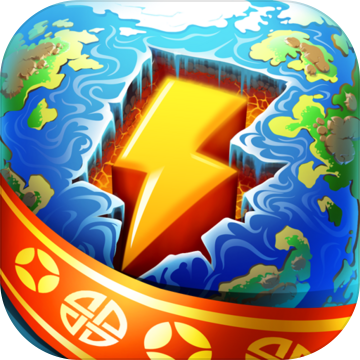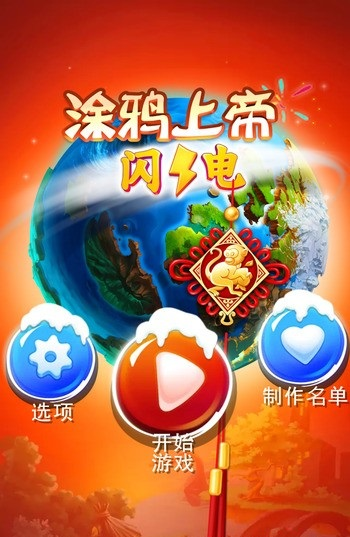7.6

涂鸦上帝：闪电中文版

• 类型:休闲益智
• 版本:v1.2.1
• 平台:安卓
• 语言:中文
• 更新:2021/10/29 17:19:47

涂鸦上帝闪电中文版特色1 能量 = 火 + 风

2 生命 = 能量 + 沼泽

3 电 = 金属 + 能量

4 蛋 = 生命 + 石头

5 种子 = 生命 + 沙子

6 性 = 人 + 人

7 血 = 人 + 恐龙

8 毒药 = 工具 + 蝎子

1 毛 = 人 + 家畜

2 肉 = 人 + 家畜

3 奶 = 人 + 家畜

4 羽毛 = 鸟 + 猎人

5 香烟 = 纸 + 烟草

6 小麦 = 农田 + 种子

7 面粉 = 石头 + 小麦

8 生面团 = 面粉 + 水

9 面包 = 火 + 生面团

10 啤酒 = 酒精 + 面包

运输系合成表(共10种)

1 轮子 = 木头 + 工具

2 小船 = 水 + 木头

3 推车 = 木头 + 轮子

4 机车 = 推车 + 蒸汽引擎

5 汽车 = 石油 + 推车

6 飞机 = 风 + 汽车

7 大船 = 木头 + 小船

8 蒸汽船 = 大船 + 蒸汽引擎

9 马车 = 推车 + 兽

10 帆船 = 大船 + 纺织物

1 风

2 蒸汽 = 风 + 水

3 灰尘 = 风 + 土

4 暴风 = 能量 + 风

5 灰烬 = 灰尘 + 火

6 雪 = 冰 + 风

1 细菌 = 生命 + 沼泽

2 杂草 = 生命 + 水

3 蘑菇 = 杂草 + 土

4 苔藓 = 杂草 + 沼泽

5 浮游生物 = 细菌 + 水

6 蠕虫 = 浮游生物 + 土

7 甲虫 = 土 + 蠕虫

8 蝴蝶 = 风 + 蠕虫

9 贝壳 = 浮游生物 + 石头

10 蝎子 = 甲虫 + 沙子

1 水

2 酒精 = 水 + 火

3 沼泽 = 土 + 水

4 伏特加 = 水 + 酒精

5 冰 = 水 + 玻璃

1 树 = 种子 + 土

2 草 = 苔藓 + 土

3 烟草 = 火 + 草

4 芦苇 = 沼泽 + 草

5 蕨类 = 苔藓 + 沼泽

6 咖啡豆=种子+能量

7沙子+树=椰子树

• 周排行
• 总排行